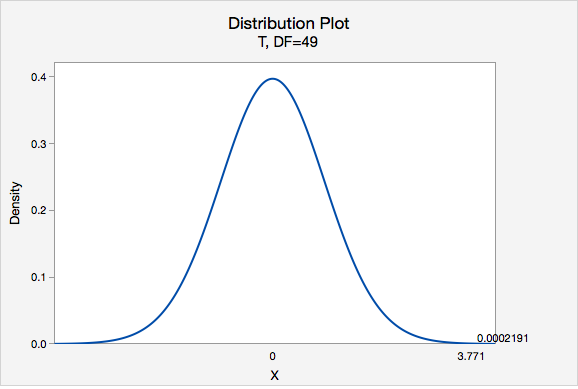# 8.2.3.1.3 - Example: Coffee

8.2.3.1.3 - Example: Coffee

In the population of Americans who drink coffee, the average daily consumption is 3 cups per day. A university wants to know if their students tend to drink more coffee than the national average. They ask a random sample of 50 students how many cups of coffee they drink each day and found $$\overline{x}=3.8$$ and $$s=1.5$$. Do they have evidence that their students drink more than the national average?

1. Check assumption and write hypotheses

Amount of coffee consumed is a quantitative variable. We are given that random sampling methods were employed. Because $$n \ge 30$$, we can approximate the sampling distribution using a t distribution.

This is a right-tailed test because we want to know if the mean in the sample is greater than the national average.

$$H_{0}:\mu= 3$$
$$H_{a}:\mu>3$$

2. Calculate the test statistic
Test Statistic: One Group Mean

$$t=\dfrac{\overline{x}-\mu_0}{\dfrac{s}{\sqrt{n}}}$$

$$\overline{x}$$ = sample mean
$$\mu_{0}$$ = hypothesized population mean
$$s$$ = sample standard deviation
$$n$$ = sample size

$$t=\dfrac{\overline{x}-\mu_0}{\dfrac{s}{\sqrt{n}}}=\dfrac{3.8-3}{\dfrac{1.5}{\sqrt{50}}}=3.771$$

Our $$t$$ test statistic is 3.771

3. Determine the p-value

$$df=n-1=50-1=49$$Using Minitab, we can find that $$P(t > 3.771) =0.0002191$$

p-value = 0.0002191

If $$\mu=3$$, then the probability of taking a random sample of $$n=50$$ and finding $$\overline{x} \geq 3$$ is 0.0002191

4. Make a decision

$$p\leq.05$$, therefore we reject the null hypothesis.

5. State a "real world" conclusion

There is evidence to state the mean number of coffees consumed in the population of all students at this university is greater than 3.

  Link ↥ Has Tooltip/Popover Toggleable Visibility